# ICSE Class 9 Maths Sample Question Paper 5 with Answers

## ICSE Class 9 Maths Sample Question Paper 5 with Answers

Section – A
(Attempt all questions from this Section)

Question 1.
(a) At what rate % p.a. will sum of ₹ 4000 yield ₹ 1324 as compound interest in 3 years ?
(a) Given : P = ₹ 4,000, C.I. = ₹  1,324, n = 3 years.
Let r be the rate % p.a.
Now A = P + C.I. = ₹ (4,000 + 1,324) = ₹ 5,324.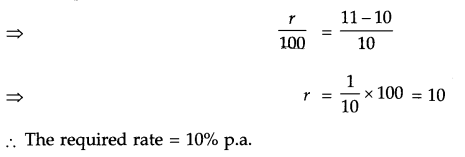(b) If x = 2 + √3 , prove that x2 – 4x + 1 = 0.(c) How many times will the wheel of a car having radius 28 cm, rotate in a journey of 88 km
Given : r = 28 cm and
Distance = 88 km = 88 x 1,000 x 100 cm = 88,00,000 cm
Now, Distance covered in 1 rotation = Circumference of wheelQuestion 2.
(a) Factorize : $$x^{2}+\frac{1}{x^{2}}-11$$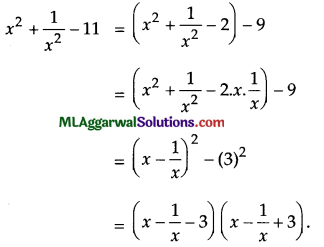(b) From the adjoining figure, find the value of x.In ΔACD,
AC = CD  (Given)
Now, ∠ADC + ∠CAD + ∠ACD = 180° (Sum of angles in a triangle is 180°)
⇒ 2∠ADC = 180° – 56°
⇒ ∠ADC $$\frac{124^{\circ}}{2}$$
∠ADC = $$\frac{124^{\circ}}{2}$$ = 62°

In ΔABD, AD = BD (Given)
∠ABD = ∠ BAD (Angles opposite to equal sides)
(Exterior angle is equal to sum of interior opposite angles)
2∠ABD =62°
∠ABD$$\frac{62^{\circ}}{2}$$=31°
In ΔABC, ∠A + ∠B + ∠ C = 180° (Sum of angles in a triangle is 180°)
x°+31°+56°=180°
x° = 180°- 87°= 93°(c) Simplify : $$\frac{5 .(25)^{n+1}-25 .(5)^{2 n}}{5 \cdot(5)^{2 n+3}-(25)^{n+1}}$$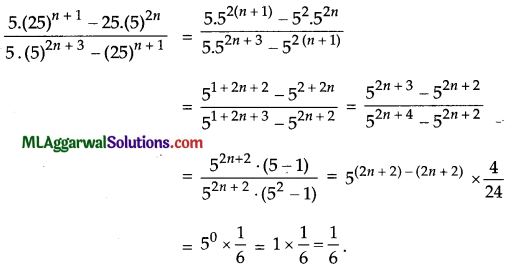Question 3.
(a) If θ = 30°, verify that cos 3 θ = 4 cos3 θ -3 cos θ .
L.H.S. = cos 3θ = cos (3 x 30°) = cos 90° = 0 ………..(1)
L.H.S. = 4cos3 θ -3cosθ= 4 cos3 30° – 3 cos 30°From (i) and (ii)
L.H.S. = R.H.S.
Hence Proved.

(b) Solve by cross multiplication method :
x – 3y – 7 = 0; 3x – 3y = 15.(c) Prove that: $$\log \frac{11}{5}+\log \frac{14}{3}-\log \frac{22}{15}=\log 7$$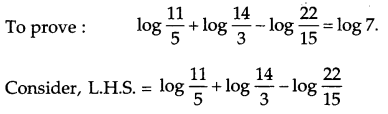= log 11 – log 5 + log 14 – log 3 – log 22 + log 15
= log 11 – log 5 + log (2 x 7)- log 3 – log (2 x 11) + log (3 x 5)
= log 11 – log 5 + log 2 + log 7 – log 3 – log 2 – log 11 + log 3 + log 5
= log 7 = R.H.S
Hence Proved.

Question 4.
(a) If a2 – 3a – 1 = 0, find the value of a + $$a^{2}+\frac{1}{a^{2}}$$
(a)(b) Construct a combined histogram and frequency polygon for the following data :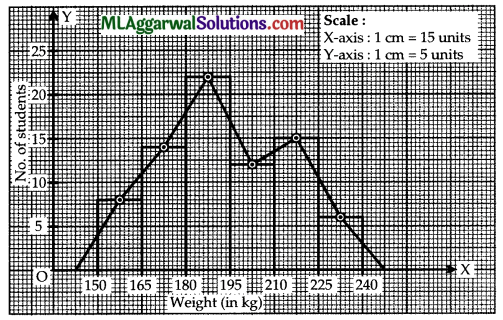(c) Of two unequal chords of a circle, prove that longer chord is nearer to the centre of the circle.
Given : AB > CD, OM⊥AB, ON ⊥ CD.
Join OA and OC.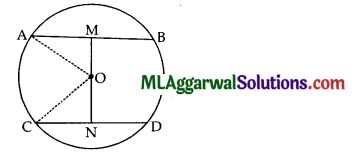We know perpendicular drawn from the centre to the chord bisects the chord.
∴ AM= $$\frac{1}{2}$$AB
and CN = $$\frac{1}{2}$$CD
Now, AM > CN(∵ AB > CD)
In ΔOAM OA2 = AM2 + OM2 (Pythagoras theorem)
In ΔOCN OC2 = CN2 + ON2 (Pythagoras theorem)
AM2 + OM2 =CN2 +ON2(∵ OA = OC, Radii)
⇒ OM2 – ON2 = – (AM2 – CN2)
⇒ OM2 – ON2 <O (∵AM > CN)
⇒ OM2 < ON2
⇒ OM < ON
i.e., longer chord is nearer to the centre.
Hence ProvedSection – B
(Attempt any four questions from this Section)

Question 5.
(a) Factorize : $$\frac{y^{6}}{343}+\frac{343}{y^{6}}$$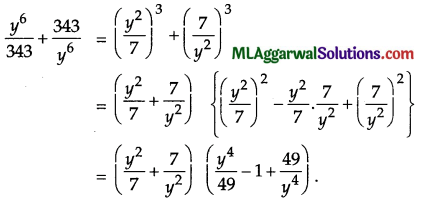(b) The diagonals AC and DB of a parallelogram intersect at O. If P is the mid-point of AD,
prove that (i) PO || AB (ii) PO = $$\frac{1}{2}$$CD.
Given : In parallelogram ABCD, diagonals AC and BD intersect at O. P is the mid-point of AD.
(i) Since diagonals of a parallelogram bisect each other
∴ O is the mid-point of DB
P and O are mid-points of sides AD and BD respectively
By mid-point theorem,(ii) Also, by mid-point theorem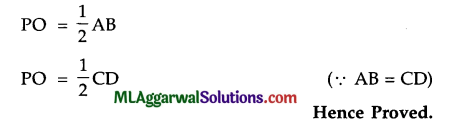(c) If θ is acute and 3sin θ = 4cos θ, find the value of 4sin2 θ – 3cos2 θ + 2.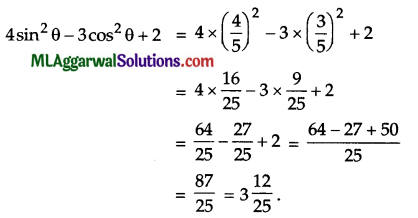Question 6.
(a) If the points A (4,3) and B (x, 5) are on the circle with centre C (2, 3), find the value of x
Given : A (4, 3), B (x, 5), C (2, 3).
∵ C (2, 3) is the centre,
∴ AC = BC (Redii)
AC2 = BC2
(4 – 2)2 + (3 – 3)2 =(x- 2) + (5 – 3)2
= 4 + 0 =(x – 2)2 + 4
(x-2)2 =0
x – 2 =0
x =2.

(b) ABCD is a trapezium with AB | | CD, and diagonals AC and BD meet at O.
Prove that area of ΔDAO = area of ΔOBC.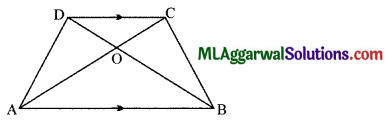Given : AB||CD, diagonals AC and BD meet at O.
AB||DC
∴  Area of ΔABD = Area of ΔABC (Triangles on same base and between same parallels are equal in area)
∴ Area of ΔDAO + Area of ΔOAB = Area of ΔOBC + Area of Δ OAB (Addition area axiom)
⇒ Area of ΔDAO = Area of Δ OBC.
Hence Proved.(c) Simplify: $$\frac{2 \sqrt{3}-\sqrt{5}}{2 \sqrt{2}+3 \sqrt{3}}$$Question 7.
(a) If x + y = 10 and x2 + y1 = 58, find the value of x3 + y3.
Given :
x + y =10, x2 + y2 = 58.
(x + y)2 = x2 + y2 + 2xy
⇒ 102 = 58 + 2xy
2xy = 100 – 58
⇒ xy = $$\frac{42}{2}$$ = 21
x3 + y3 = (x + y)3 – 3xy (x + y)
= 103 – 3 x 21 x 10
= 1000 – 630 = 370.

(b) The larger of two supplementary angles exceeds the smaller by 18°. Find the angles.
Let the larger supplementary angle be
Then, smaller supplementary angle = 180° – x According to the question,
x – (180° – x) = 18°
⇒ x – 180° + x =18°
⇒ 2x = 18° + 180°
⇒ x= $$\frac{198^{\circ}}{2}$$ = 99°
∴ 180° –  x = 1800 99° = 81°
The supplementary angles are 990 and 81°.(c) The mean of 5 numbers is 20. If one number is excluded, mean of the remaining numbers becomes 23. Find the excluded number.
Mean of 5 numbers = 20
∴Sum of 5 numbers = 20 x 5 = loo.
1f one number is excluded,
Then, Mean of 4 numbers = 23
Sum of 4 numbers = 23 x 4 = 92
The excluded number = 100 – 92 = 8.

Question 8.
(a) Solve for x : 9 x 3X = (27)2x-5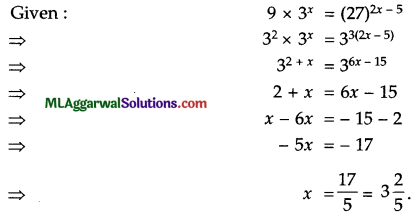(b) In a right-angled triangle, if hypotenuse is 20 cm and the ratio of the other two sides is
4 : 3, find the sides.
Given: Hypotenuse = 20 cm
and ratio of the other two sides = 4:3
Let the other two sides be 4x and 3x.
∴ By Pythagoras theorem,4x – 4 x 4 = 16
3x = 3 x 4 = 12
∴ The required sides are 16 cm and 12 cm.

(c) Without using tables, find the value of :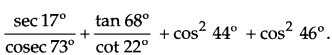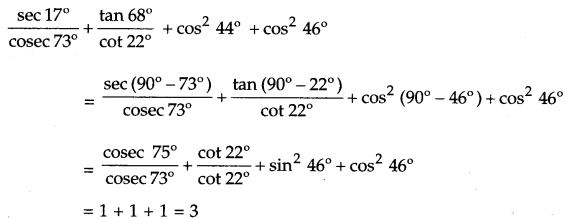Question 9.
(a) In what time will a sum of ₹ 8000 becomes ₹ 9261 at the rate of 10% p. a., if the interest is compounded semi-annually?
Given : P = X 8,000, A = ? 9,261, r = 10% p.a.
Let n be the number of years.
∵ C.I. is compounded semi-annually,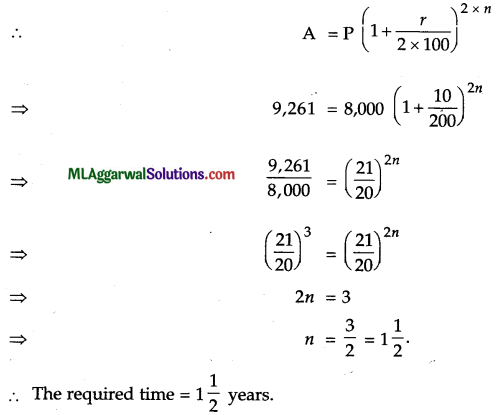(b) Construct a regular hexagon of side 2.2 cm.
Each side = 2.2 cm.Steps of construction :
(1) Draw AB 2.2 cm
(2) At A and B, draw angle of 120°.
(3) From A and B, cut-off arcs of 2.2 cm each.
(4) At C, draw 120° and cut it off at D so that CD = 2.2 cm.
(5) At D, draw 120° and cut-off DE = 2.2 cm.
(6) Join EF.
Then, ABCDEF is the required hexagon.(c) Solve graphically : 2x – 3y + 2 = 4x + 1=3x-y + 2
Given: 2x – 3y + 2 = 4x + 1=3x-y + 2
∴ 2x – 3y + 2 = 4x + 1 and 4x + 1= 3x-y + 2
⇒ 4x – 2x = – 3y + 2 – 1 and 4x-3x = -y + 2- 1
⇒  2x = 1 – 3yThe two lines intersect at the point (2, – 1).
x =2, y = -1

Question 10.
(a) Express (x2 -5x + 7) (x2 + 5x – 7) as a difference of two squares.
(x2 – 5x + 7) (x2 + 5x – 7) = {x2 – (5x – 7)} {x2 + (5x – 7)}
= (x2)2 – (5x – 7)2(b) Simplify: $$(64)^{2 / 3}-\left(\frac{1}{81}\right)^{-1 / 4}+8^{2 / 3} \cdot\left(\frac{1}{2}\right)^{-1} \cdot 3^{0}$$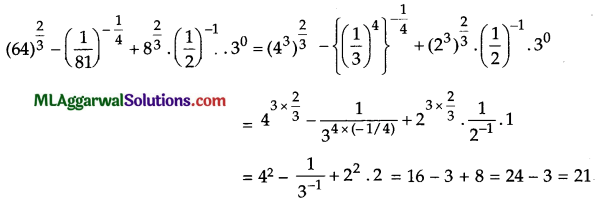(c) In a pentagon ABCDE, BC | | ED and ∠B: ∠A: ∠E = 5:3:4. Find ∠B.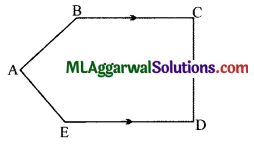Given: ∠B: ∠A: ∠E =5:3:4
Let Now, ∠B = 5x, ∠A = 3x, ∠E = 4x.
∠C + ∠D = 180°(Co-interior angles; BC||ED)
Sum of angles in a figure with number of sides ‘n’ = (n – 2) x 180°
In pentagon, sum of angles = (5 – 2) x 180°
= 3 x 180° = 540°
∴ ∠ A + ∠B + ∠C + ∠D + ∠E = 540°
⇒ 3x + 5x + 180° + 4x = 540°
⇒ 12x = 540° – 180°
⇒ $$x=\frac{360^{\circ}}{12}$$
⇒ x = 30°
∴ ∠B = 5x = 5 x 30° = 150°

Question 11.
(a) If p + q = 10 and pq = 21, find 3 (p2 + q2).
p + q = 10, pq = 21.
∴ p2 + q2 = (p + q)2 – 2pq = 102 – 2 x 21 = 100 – 42 = 58
3 (p2 + q2) = 3 x 58
= 174.(b) Find the area of an isosceles triangle whose base is 6 cm and perimeter is 16 cm
Let length of each of equal sides be a and that of base be b.
b  = 6 cm(Given)
and Perimeter   = 16 cm
⇒ a + b  = 16
⇒ 2a   + 6  = 16
⇒ 2a = 16 – 6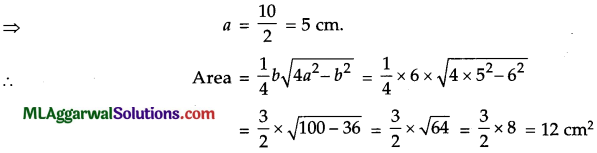(c) Prove that : $$\frac{1}{1+\tan ^{2} \theta}+\frac{1}{1+\cot ^{2} \theta}=1$$